# Translating and Scaling in Graphing Modified Functions

Go back to  'Functions'

[f]     $$\boxed{y = f\left( x \right)\,\,{\text{to}}\,y = f\left( x \right) \pm k}$$

Here, we are adding (or subtracting) ‘$$k$$’ units to the value of $$y$$ for every point $$x,$$ or in other words, we are incrementing or decrementing the value of ‘$$y$$’ by the same amount everywhere. For a graph, we are shifting it upwards or downwards while exactly retaining its shape.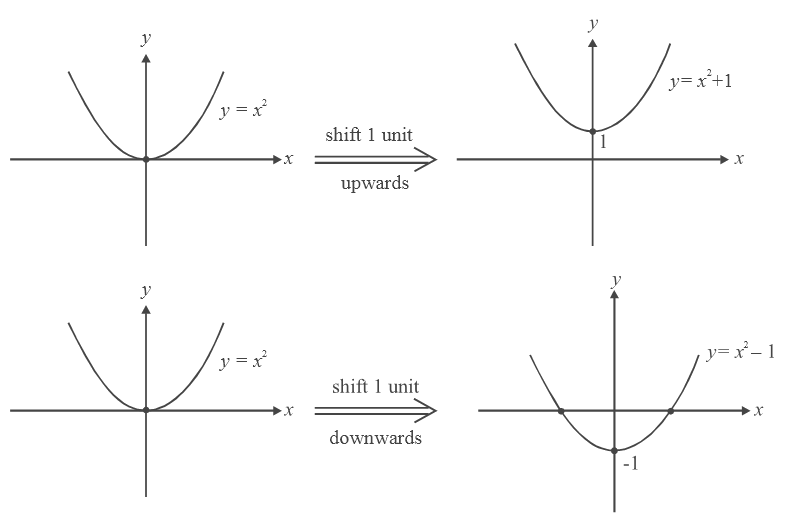Draw the graphs of $$\left[ x \right] \pm 1,\,\,\,\left\{ x \right\} \pm 2,\,\,\,{\rm{and}}\,\,\,\sin x \pm \frac{1}{2}$$ by yourself.

[g]     $$\boxed{y = f\left( x \right)\,\,{\text{to}}\,\,y\, = f\left( {x \pm k} \right)}$$

Recall that we’ve discussed one such case earlier where we drew the graphs of y =\begin{align} \frac{1}{{x \pm 1}}\end{align} from the graph of y = \begin{align}\frac{1}{x}\end{align} .

This is elaborated again here.

Consider the graph of $$y = f (x).$$ Assume a particular value for $$x$$ say  $$x{_0}$$ and for this value, suppose $$y$$ takes the value $$y{_0}.$$ Therefore $$y{_0}$$ = f($$x{_0}.$$) Now consider $$y = f (x + k).$$ For what value of $$x$$ will I get the output as $$y{_0}?$$ Obviously, $$x = {x_0} - k,$$ because $$y{_0}$$ = f ($$x{_0}$$) = f (($$x{_0}$$    – k ) + k.) Hence, the output $$y{_0}$$    will now come ‘k’ units earlier on the graph. This argument is true for each value of x (since $$x{_0}$$     is arbitrary here). Hence the entire graph will appear ‘k’  units earlier on the axes, or, as is standard terminology, advanced, k units to the left. Similarly, the graph of f (x–k) will be delayed, k units to the right. Obviously, if k is negative, f (x+k ) is actually shifted to the right and f (x–k) to the left. Some examples will make this clear.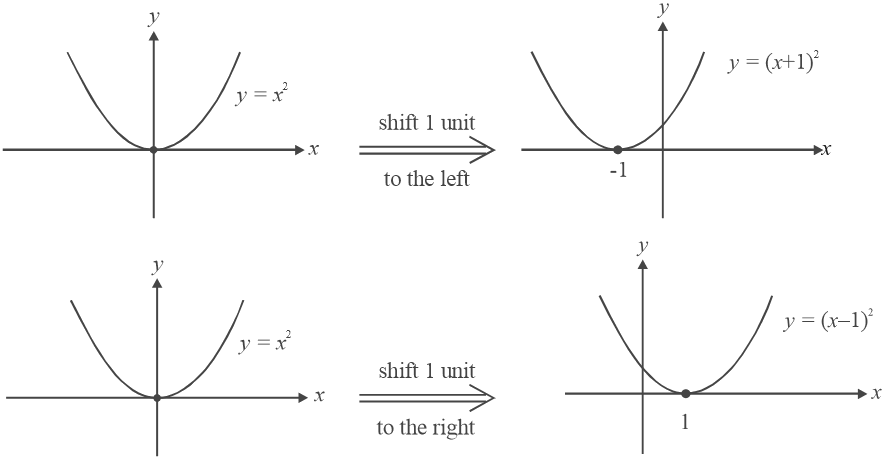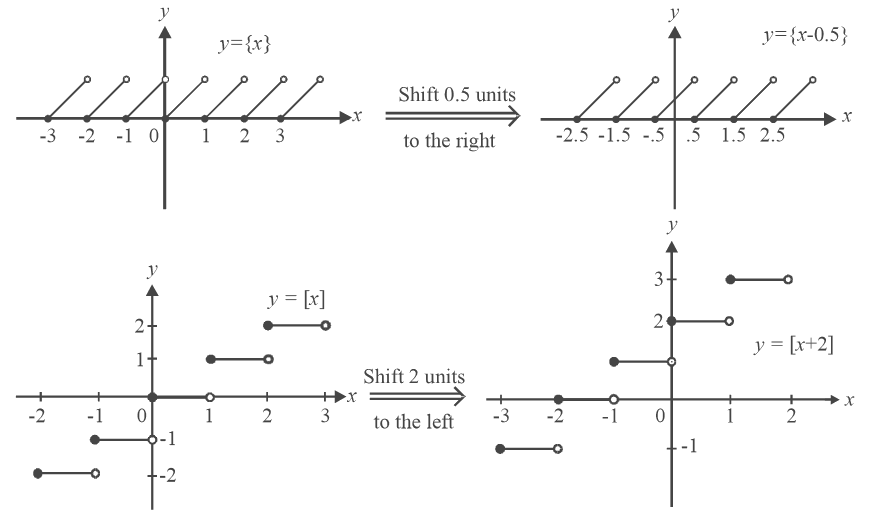[h]     $$\boxed{y = f\left( x \right)\,\,{\text{to}}\,\,y = k\,f\left( x \right)}$$

Suppose $$y = x{^2}$$ is our original graph and we want to draw the graph for $$y{\rm{ }} = 2x{^2}$$ . What is happening? The value for y is increasing (being doubled every where). But this increase is not uniform. Hence there is no uniform upwards or downwards shift. Also, we can see that there is no left or right shift. We can infer that the graph does change but not in the form of a ‘shift’.  It ‘stays’ where it is. If y = 0 for some $$x{_0}$$    on the graph  y = f (x), it is also 0 for the graph y = kf (x). The zeroes of the function (or the graph) remain the same. Every where else, y increases or decreases in magnitude depending on whether $$\left| k \right| > \,\,1\,\,{\rm{or}}\,\,\,\,\left| k \right| < 1$$.

We can say that the graph expands or compresses in the  y – direction.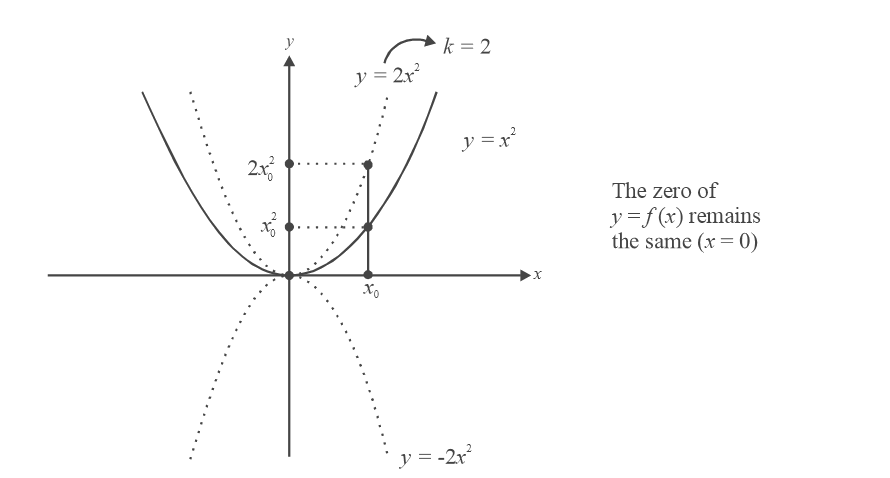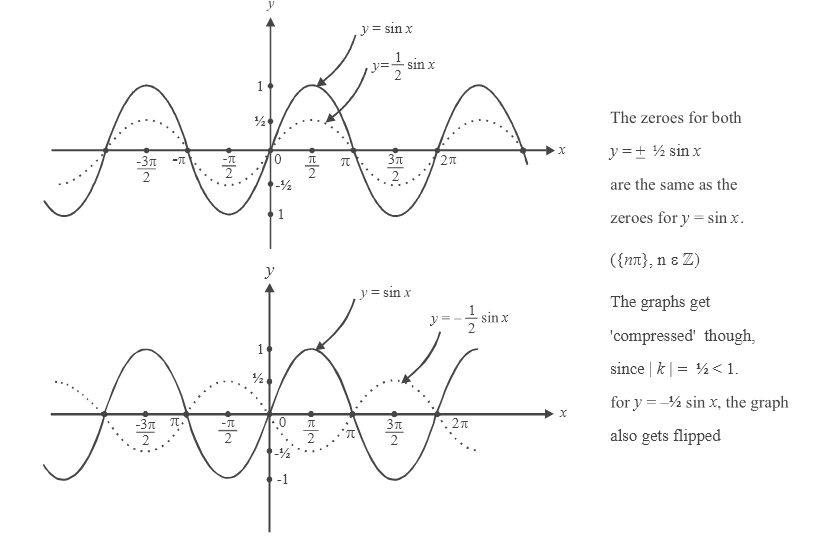If it is helpful, think that some fictitious mathematical creature is stretching or compressing the graph of   y = f (x) to obtain the graph of y = k f (x), depending on the value of k (This creature will also be flipping the graph about the x–axis if k < 0.)

Draw the graphs of $$\pm 2\left[ x \right],\,\,\,\, \pm \frac{1}{2}\{ x\}$$ yourself.

[i]     $$\boxed{y = f\left( x \right)\,\,{\text{to}}\,\,y = f\left( {kx} \right)}$$

Lets consider a particular value of k to make things easier. Take k = 2. For our original function, suppose $$x{_0}$$  gives the output $$y{_0}$$ , that is, $${y_0} = f\left( {{x_o}} \right)$$  . In the new function, y = f (2x), at what value of x will the output be $$y{_0}$$ ? Obviously, at   $${x_0}/2$$  since $$y{_0}$$  = f ($$x{_0}$$) = $$f\left( {2\left( {\frac{{{x_0}}}{2}} \right)} \right)$$   .  Hence, as in the case        y = f (x + k) where the same output came k units earlier, in  y = f (2 x), the same output will come at half the original value: our mathematical creature will compress the graph along the x-axis ‘by a factor of 2’.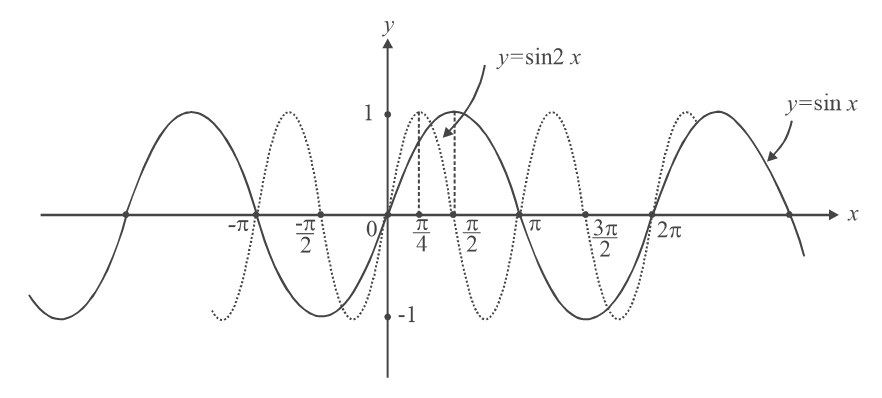What will happen if k = 1/2? Our being will stretch the graph by a factor of 2.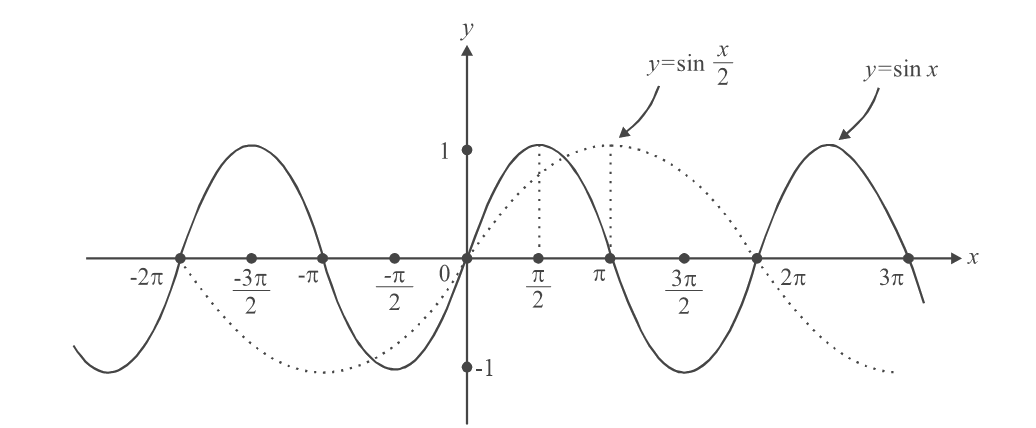What will happen if k = –2? Our being will first compress the graph by a factor of 2 and then flip it about the y–axis (see part b). Or he can flip it first and then compress it.

$\left\{ {f(x) \to f(2x) \to f( - 2x)\,\,\,\,\,\,\,{\rm{or}}\,\,\,\,\,f(x) \to f( - x) \to f( - 2x)} \right\}$

Try drawing the graphs of  \begin{align} \cos \left( {\frac{x}{{ \pm 3}}} \right),\,\left[ {2x} \right],\,\,\,\log \,\left( {\frac{x}{5}} \right)\end{align}

## SUMMARY

 (1)$$y{\rm{ }} = {\rm{ }}f\left( x \right)\;to\;{\rm{ }}y{\rm{ }} = -f\left( x \right)$$ : Flip about the x – axis (2) $$y{\rm{ }} = {\rm{ }}f\left( x \right)\;to\;y{\rm{ }} = {\rm{ }}f\left( {-x} \right)$$ : Flip about the y – axis (3) $$y = f(x)\;to\;{\rm{ }}y = {\rm{ }}\left| {f\left( x \right)} \right|$$ : Reflect the parts of the graph that lie in the lower  half (negative parts) into the upper half of the    axes. (4)  $$y = f(x)\;to\;{\rm{ }}y = f\left( {\left| x \right|} \right)$$ : Discard the left part of the graph (for x < 0) and take a reflection of the right part of the graph into the left half of the axes. (5)$$y = f\left( x \right)\,\,{\rm{to}}\,\,\left| y \right|\, = \,f\left( x \right)$$ : Discard the lower part of the graph ( f (x) < 0 ) and  take a reflection of the upper part of the graph into the lower half of the axes. (6)$$y{\rm{ }} = {\rm{ }}f\left( x \right)\;to\;y{\rm{ }} = {\rm{ }}f\left( x \right) + k$$ : Shift the graph $$\,\left| k \right|\,$$  units upwards or downwards depending on whether k is  positive or negative respectively. (7)$$y{\rm{ }} = {\rm{ }}f\left( x \right)\;to\;y = f\left( {x + k} \right)$$ : Advance (shift left) or delay (shift right) the graph by $$\,\left| k \right|\,$$ units depending on whether k is positive or  negative respectively. (8) $$y = f\left( x \right)\;to\;y = kf\left( x \right)$$ :  Stretch or compress the graph along the y – axis Depending on whether $$\,\left| k \right|\, > 1\,\,\,{\rm{or}}\,\,\,\,\left| k \right|\, < 1$$ respectively.Also, flip it about the x-axis if k is negative (This latter statement follows from part 1) (9) $$y = f\left( x \right)\;to\;y = f\left( {kx} \right)$$ : Stretch or compress the graph along the x-axis  depending on whether  $$\,\left| k \right|\, < 1\,\, {\rm{or}}\,\,\,\left| k \right|\, > 1\,\,$$ respectively. Also flip it about the y–axis if k is  negative (The     latter statement follows from part 2)

These rules summarize all that you need to know about plotting graphs for the time being. A knowledge of limits and derivatives will make graph plotting more accurate, but for now, lets plot some graphs using what we’ve learnt upto this point.